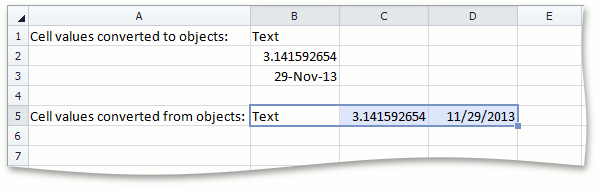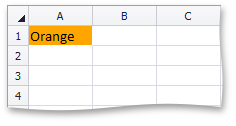21.1
20.2
20.1
19.2
19.1
18.2
18.1
17.2
A newer version of this page is available. Switch to the current version.

# How to: Convert Objects to Cell Values and Cell Values to Objects

• 4 minutes to read
• Convert Cell Values To⁄From Objects

The Range.SetValue and CellValue.FromObject methods allow you to convert objects of different types to SpreadsheetControl-compatible cell values. The CellValue.ToObject method performs an inverse operation - it gets objects from cell values.

The code below demonstrates how to convert cell values of different types to objects and add them to an array, and convert array elements to CellValue objects and assign them to cells. In this example, the default cell value converter is used. However, you can create your own custom converter that implements the ICellValueConverter interface and use it for conversion.

##### NOTE

A complete sample project is available at https://github.com/DevExpress-Examples/winforms-spreadsheetcontrol-api-e4655

``````// Add data of different types to cells of the range.
Range sourceRange = worksheet["B1:B3"];
sourceRange.Value = "Text";
sourceRange.Formula = "=PI()";
sourceRange.Value = DateTime.Now;
sourceRange.NumberFormat = "d-mmm-yy";

// Get the number of cells in the range.
int cellCount = sourceRange.RowCount * sourceRange.ColumnCount;

// Declare an array to store elements of different types.
object[] array = new object[cellCount];

// Convert cell values to objects and add them to the array.
for (int i = 0; i < cellCount; i++) {
array[i] = sourceRange[i].Value.ToObject();
}

// Convert array elements to cell values and assign them to cells in the fifth row.
for (int i = 0; i < array.Length; i++) {
worksheet.Rows["5"][i + 1].SetValue(array[i]);
// An alternative way to do this is to use the CellValue.FromObject method.
// worksheet.Rows["5"][i+1].Value = CellValue.FromObject(array[i]);
}
``````

The following image shows the result of executing the code above. Cell values from the source range (B1:B3) are converted to array elements. These elements are then converted back to cell values and assigned to cells in the fifth row.• Use a Custom Converter

To convert custom objects to cell values and vise versa, you can apply your own converters.

This example demonstrates how to convert a color object (Color) to a SpreadsheetControl-compatible cell value of the text type (CellValue) that corresponds to the color name. To do this, create a custom converter class that implements the ICellValueConverter interface, and call the CellValue.FromObject method with the color object and custom converter passed as parameters.

##### NOTE

A complete sample project is available at https://github.com/DevExpress-Examples/winforms-spreadsheetcontrol-api-e4655

``````        Worksheet worksheet = workbook.Worksheets;
Cell cell = worksheet.Cells["A1"];
cell.FillColor = Color.Orange;
cell.Value = CellValue.FromObject(cell.FillColor, new ColorToNameConverter());
// ...
class ColorToNameConverter : ICellValueConverter {
object ICellValueConverter.ConvertToObject(CellValue value) {
return null;
}
CellValue ICellValueConverter.TryConvertFromObject(object value) {
bool isColor = value.GetType() == typeof(Color);
if (!isColor)
return null;
return ((Color)value).Name;
}
}
``````

The following image shows a cell with a value converted from its color.See Also